# How to Use Google Sheets Weekday Function With Example

Weekday Function in Google Sheets: When working with Google Sheets, we might tend to have To-Do Lists along with dates. In some instances, we might also want to know the weekday for the specified dates. This is where Google Sheets Weekday Function comes into the picture. The weekday function in google sheets is helpful for knowing the day of the week, for a given date.

## Google Sheets Weekday Function Syntax

Below is the syntax for using weekday function in google sheet,

=WEEKDAY(date, [type])

• WEEKDAY – is the keyword for using this function
• date – is the input date that the function takes for knowing the day of the week. This can be a reference to a number that the Google sheets can evaluate to a date.
• type – this is optional that lets the function know where to begin the week from. This parameter will hold numeric values (1, 2, and 3)
• 1 is the value by default. Here the week starts from Sunday and ends on Saturday. The value 1 is Sunday and value 7 is Saturday.
• If we consider 2 as a parameter, the week begins on Monday and ends on Sunday. The value for Monday is 1 and the value of Sunday is 7
• If we consider 3 as a parameter, the week begins on Monday and ends on Sunday. The only difference with the above parameter is, the value for Monday is 0 and Sunday is 6

## How to Use the Weekday Function to Get the Weekday as Number?

Below are a few examples for a better understanding of the Google Sheets Weekday function,

### Example 1: To Find the Weekday With Type 1

In Google Sheets, we are using the following formula to find out the weekday using Type 1:

=WEEKDAY(“3/7/1994”)

Here by default, the type will be considered as 1 and the day starts with Sunday and ends on Saturday.In the above example, the date March 7th, 1994 is Monday which would give us 2 (as Sunday is assigned to 1 and Monday is assigned to 2  and so on).

### Example 2: To Find the Weekday With Type 2

In Google Sheets, we are using the following formula to find out the weekday using Type 2:

= WEEKDAY(“3/7/1994”,2)

Here in type 2, the day starts with Monday and ends on Sunday.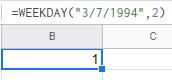In the above example, the date March 7th, 1994 is Monday which would give us 1 as a result (as Monday is assigned to 1 and Tuesday is assigned to 2 and so on)

### Example 3: To Find the Weekday With Type 3

Here in type 3, the day starts with Monday as 0 and ends on Sunday as 6.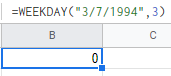In the above example, the date March 7th, 1994 is Monday which would give us 0 as a result ( as Monday is assigned to 0 and Tuesday is assigned to 1 and so on)

### How to Use the Weekday Function to Find Out the Weekday Name or a Specific Date?

Since the weekday function returns the weekday with numbers it is still confusing for people. To know the exact weekday name or specific date, we can call the WEEKDAY function with CHOOSE function.

Example 1: To know what weekday on March 7th, 1994, you can use the below WEEKDAY function affixed with CHOOSE function in Google Sheets,

=CHOOSE(WEEKDAY(DATE(1994,3,7),2),”Mon”, “Tue”, “Wed”, “Thu”, “Fri”, “Sat”, “Sun”)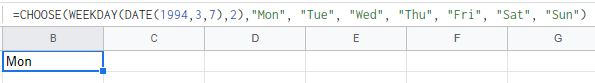In the above example, we get the weekday name instead of the weekday number which is user-friendly.

Also here, the WEEKDAY function returns the number, and CHOOSE function converts into the day(weekday name).

Example 2: To find the first Monday of the month, you can use the below format,

Here we will find out the first Monday in June 2018. In order to find out the first Monday in June 2018, use the following WEEKDAY formula in Google Sheets:

=IF((WEEKDAY(DATE(2018,6,1),2))>1,DATE(2018,6,1)+7(WEEKDAY(DATE(2018,6,1),2))+1,DATE(2018,6,1))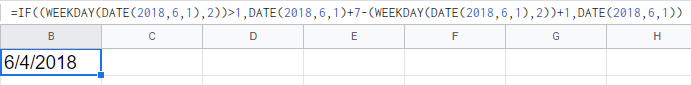This will return to us first Monday of June 2018, i.e., 4th June 2018.

### EOMONTH Function in Google Sheets

EOMONTH – End Of Month. This function finds the last date of a given month.

Example 1: To find the last Monday of the month, you can use the below format,

Here we will find out the last Monday in May 2018,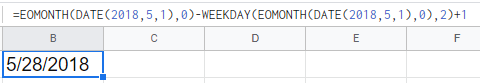This will return to us last Monday of May 2018, i.e., 28th May 2018.